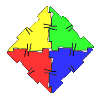#### You may also like### Polydron

This activity investigates how you might make squares and pentominoes from Polydron.If you had 36 cubes, what different cuboids could you make?### Cereal Packets

How can you put five cereal packets together to make different shapes if you must put them face-to-face?

# Penta Primes

##### Age 7 to 11Challenge Level

You have sent in some very well thought out answers to this problem, thank you. Some of you decided to include numbers with a zero at the beginning (e.g. $05$ rather than $5$), some of you ignored these. The question didn't make this clear, but I think we will stick to the usual way of writing numbers i.e. with no leading zero.

Kirsty from Tattingstone School knew that she couldn't use prime numbers with more than one digit the same. So, she tried combinations of the ten different digits, crossing them out on a list so as not to use one more than once. This is a sensible strategy, Kirsty. She found nine ways:

 431 809 67 5 2 401 829 67 3 5 709 683 41 5 2 601 829 43 5 7 607 839 41 5 2 701 463 89 5 2 409 683 71 5 2 461 307 89 5 2 467 103 89 5 2

Bronya also from Tattingstone explained that she tried to work out combinations of digits in a similar way. She found two other solutions:

$487, 601, 59, 2, 3$ and $67, 89, 41, 503, 2$

Donovan who lives in Castle Rock, Colorado, USA sent us an email saying that he has written a programme on his computer to find all the possible solutions to Penta Primes. I asked him how he went about writing the programme and he explained:

The program has four main parts to it.

### Step 1

I had it look through all combinations of ten-digit strings of numbers: $0000000000$ to $9999999999$. It extracted out only the ten-digit strings where each single digit was used just once. This gave me a list of $3628800$ ten-digit strings out of $10$ billion total.

### Step 2

Then I had it go through all seven combinations of digit sizes for the five numbers. Here are the seven sets of different digit sizes:

$1-1-1-1-6$
$1-1-1-2-5$
$1-1-1-3-4$
$1-1-2-2-4$
$1-1-2-3-3$
$1-2-2-2-3$
$2-2-2-2-2$

Then it created the five numbers for each of the seven-digit sizes on each of the $3628800$ ten-digit strings.

Example: If the ten-digit string the program was looking at is $9876543210$ and the number sizes were $1-1-1-3-4$, then the five numbers it would create would be: $9, 8, 7, 654, 3210$. I saved the list of all these sets of five numbers it created. This gave me lots of duplicates, so I had it sort this list and deleted out all the duplicates.

### Step 3

Then the program calculated all prime numbers less than a million and saved them in a lookup table.

Example: Position $23$ in the lookup table would be set to one because it is prime. Position $25$ would be set to zero because it is not a prime.

### Step 4

Then the program looked through all the sets of five numbers created in step $2$. It looked for which ones had all five numbers prime using the lookup table created in step $3$. This created a list $1030$ sets of five numbers. Then I had it delete out the ones that had a number with a leading zero. This left $540$ solutions to the problem.

Thank you for sharing this with us Donovan. While some of us may not be able to write the computer programme, we can understand the mathematical thinking that you went through which is very valuable.